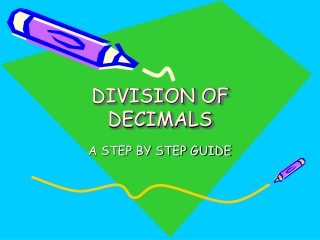DownloadDownload PresentationDIVISION OF DECIMALS

# DIVISION OF DECIMALS

Télécharger la présentation## DIVISION OF DECIMALS

- - - - - - - - - - - - - - - - - - - - - - - - - - - E N D - - - - - - - - - - - - - - - - - - - - - - - - - - -
##### Presentation Transcript

1. DIVISION OF DECIMALS A STEP BY STEP GUIDE

2. STEP 1- SETTING UP THE DECIMALS Start out by setting up as a long division problems 2 Quotient 8 16 Dividend Divisor

3. YOUR TURN SET UP THE FOLLOWING PROBLEMS IN LONG DIVISION FORM #1. 144 ÷ 2 #2. 16 ÷ 8

4. SOLUTIONS #1- 144 divided by 2 2 144 #2- 16 divided by 8 8 16 72 2

5. STEP 2-FINDING YOUR DECIMAL PLACE When you divide a decimal you have to move the decimal in the divisor to the right as many times as necessary to make it a whole number! If you move the decimal in the divisor 3 times then you have to move the decimal in the dividend 3 times!

6. Examples 1.54.5 Move your decimal over once on both the the dividend and divisor so it looks like…

7. Examples 15. 45. Move your decimal over once on both the the dividend and divisor so it looks like this

8. STEP 3-PLACE YOURDECIMAL IN THE QUOTIENT Now find your decimal place in the dividend pop it up directly above into the quotient spot- Now Divide! 3. 15 45. 15 45 .

9. LET’S DO SOME MORE EXAMPLES! .003 1.2001.2 60 SOLUTIONS TO EXAMPLES 400. 5 .0031.200 1.2 60

10. WHAT HAPPENS WHEN… You run out of spaces to put your decimal- simple you add zeros to your dividend 002 04 . . 0

11. 204 0 . NOW YOU DIVIDE!

12. ANSWER! .002 ÷ .04 = 20

13. EXAMPLE PROBLEMS 1.2 .36 .007 .21 SOLUTIONS TO EXAMPLES 1 & 2 1.2 .36 = .3 .007 .21 = 30

14. If you need more help… Go to the following website! http://www.bmcc.org/nish/MathTutorials/Decimals/decdiv.htm

15. \ CONGRATULATIONS! YOU HAVE COMPLETED THE MULTIPLICATION AND DIVISION OF DECIMALS TUTORIAL! YOU SHOULD NOW UNDERSTAND THE CONCEPTS!

16. STES